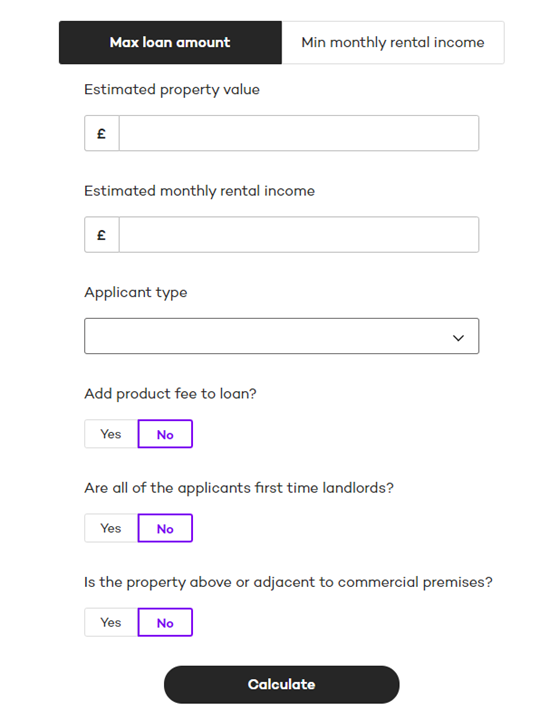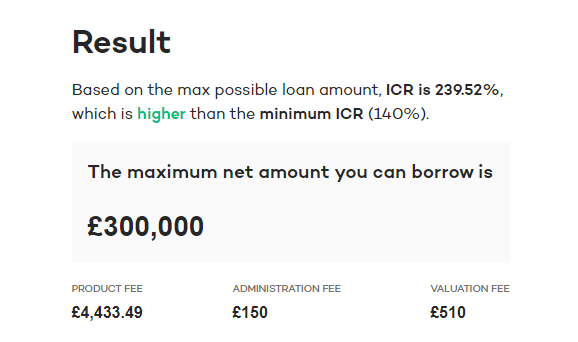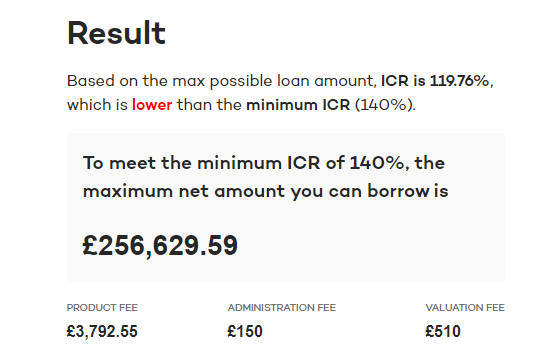1. Knowledge base
2. Products and BTL Calculator

# How do you calculate max loan amount?

## The max loan amount calculator checks what you can borrow based on your rental income and the product's maximum loan size and LTV1. The property value is checked against Landbay’s minimum
2. The maximum possible loan amount is calculated based on the max LTV and max loan amount.
• E.g., If the property value is £1,000,000 and the max LTV is 80%, the max possible loan amount is £800,000
• If the product has a max loan amount of £500,000, this is the max possible loan amount as it is the lower of the two values
3. If product fee is added to loan, gross loan amount is calculated as max possible loan + product fee, otherwise it is just the max possible loan amount
4. ICR is calculated based on the above gross loan amount and estimated monthly rental income
• Monthly rental income / ((gross loan amount * stress rate) / 12)
5. If the ICR is above the minimum required for the case, the applicant can borrow the maximum possible amount calculated in step 26. If the ICR does not meet the minimum, the maximum loan amount is calculated based on the estimated monthly rental income:
• (Monthly rental income * 12) / Minimum ICR / Stress rate
• If product fee was added to the loan, it is subtracted
7. This value is checked against the minimum loan amount for the product8. The value returned is the net loan amount, as this is the amount you can borrow against the property and is the value to be entered into an application. If opting to add the product fee to the loan, this is factored in.